# Paul Balister and Michael Savery (University of Oxford) : Improved bounds for 1-independent percolation on $Z^n$

Abstract:

A 1-independent bond percolation model on a graph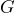is a probability distribution on the spanning subgraphs ofin which, for all vertex-disjoint sets of edges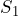and, the states (i.e. present or not present) of the edges inare independent of the states of the edges in. Such models typically arise in renormalisation arguments applied to independent percolation models, or percolation models with finite range dependencies. A 1-independent model is said to percolate if the random subgraph has an infinite component with positive probability. In 2012 Balister and Bollob\’as defined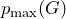to be the supremum of those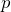for which there exists a 1-independent bond percolation model onin which each edge is present in the random subgraph with probability at leastbut which does not percolate. A fundamental and challenging problem in this area is to determine, or give good bounds on, the value ofwhenis the lattice graph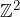. Since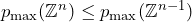, it is also of interest to establish the value of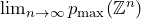.

In this talk we will present a significantly improved upper bound for this limit as well as improved upper and lower bounds for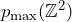. We will also show that with high confidence we have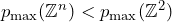for large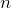and discuss some open problems concerning 1-independent models on other graphs.

This is joint work with Tom Johnston and Alex Scott.

______________________

Based on

## Video